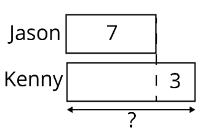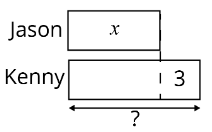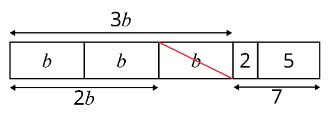Study P6 Mathematics Algebra - Geniebook# Algebra

Algebra involves the representation of numbers using letters, such as $$a, b, c, x, y, z$$ etc. We use letters to represent unknown numbers.

In this article, we are going to look at what we need to know for algebra:

1. Using letters to represent unknown numbers
2. Algebraic rules for multiplying and dividing
3. Simplifying simple algebraic expressions
4. Evaluating simple algebraic expressions

## 1. Using letters to represent unknown numbers

Question 1a:

Jason is $$3$$ years younger than Kenny.

(a) If Jason is $$7$$ years old, how old is Kenny?

Solution:Jason’s age $$= 7$$ years old

Kenny’s age $$= 7+3$$

$$= 10$$ years old

$$10$$ years old

Question 1b:

(b) If Jason is $$x$$ years old, how old is Kenny?

Solution:Jason’s age $$= x$$ years old

Kenny’s age $$= (x+3)$$ years old

$$(x+3)$$ years old

Note: Remember to put a pair of brackets for algebraic expressions with units as the units belong to the whole expression.

Question 2:

Peter bought $$y$$ apples. He ate $$4$$ apples. How many apples had he left in terms of $$y$$?

Solution:

Number of apples left $$= (y-4)$$ apples

$$(y-4)$$ apples

Did you observe that algebraic terms and numbers cannot be simplified further? This is because they are called unlike terms. Only algebraic terms of the same kind can be grouped together. You will learn more about this below.

## 2. Algebraic Rules for Multiplying and Dividing

1. For algebraic expressions involving multiplication, do not write the multiplication sign. For example:

\begin{align} 4 \times y &= 4y \\ 7 \times x &= 7x \\ b \times 6 &= 6b \end{align}

1. For algebraic terms, numbers are always in front of the letters. For example:

\begin{align} 9 \times y &= 9y \;\;\text{ not }\;\; y9\\ a \times 8 &= 8a \;\;\text{ not }\;\; a8 \end{align}

1. For the product of $$1$$ and any letter, the number $$1$$ is not written. For example:

\begin{align} 1 \times y &= y \\ a \times 1 &= a \end{align}

1. For algebraic expressions involving division, do not write the division sign. For Example:

\begin{align} 8y \div 5 &= \frac {8y}{5} \\ 9a \div 2 &= \frac {9a}{2} \\ 7 \div 3b &= \frac {7}{3b} \end{align}

The first number in the division statement is the numerator while the second number is the denominator.

Note: Take note of the last example, $$3b$$ is written as the denominator. The expression $$\frac {7}{3b}$$ is not the same as $$\frac {7}{3}\small b$$ or $$\frac {7b}{3}$$. Think about it, why?

Question 1:

There are $$4$$ bags. If there are $$m$$ books in each bag, how many books are there altogether?

Solution:

\begin{align} \text{Total number of books} &= 4\times m\\ &=4m \end{align}

$$4m$$ books

Question 2:

What is the product of $$3$$ and $$b$$?

Solution:

\begin{align} \text{Product of 3 and }b &= 3\times b\\ &=3b \end{align}

$$3b$$

Question 3:

Gary bought some chocolate bars for $$7$$ students. He gave the same number of chocolate bars to each student. If he bought $$w$$ chocolate bars, how many chocolate bars would each student receive?

Solution:

\begin{align} \text{Number of chocolate bars each student receive} &= w\div 7\\ &=\frac {w}{7} \end{align}

$$\frac {w}{7}$$ chocolate bars

## 3) Simplifying Algebraic Expressions

We can only add or subtract algebra of the same letters. For example:

\begin{align} 5a - 3a &= 2a \\ 5b - 8 &= (5b-8) \end{align}

Note: Terms that do not have the same letters cannot be simplified. Only algebraic terms of the same kind can be simplified by addition or subtraction. We called them like terms.

To simplify algebraic expressions:

Step 1:

Identify all the terms with the same letters first

Step 2:

Rearrange them (remember the sign belongs to the term behind it)

Step 3

Add or subtract algebra of the same letters or like terms

Question 1:

Simplify $$3b+2-b+5$$

Solution:\begin{align} &3b+2-b+5\\ &= 3b-b+2+5 \\ &=2b+7 \end{align}

$$2b+7$$

Question 2:

Simplify $$6a+10-2a-3$$

Solution:

\begin{align} &6a+10-2a-3\\ &= 6a-2a+10-3 \\ &=4a+7 \end{align}

$$4a+7$$

Question 3:

Simplify $$10w-3-5w+13+3w$$

Solution:

\begin{align} &10w-3-5w+13+3w\\ &=10w-5w+3w-3+13 \\ &=8w+10 \end{align}

$$8w+10$$

## 4) Evaluating Simple Algebraic Expressions

To evaluate algebraic expressions,

Step 1:

Substitute the letter by a given value.

Step 2:

Question 1:

Evaluate the following equation given that $$a=3$$ .

$$5a+13$$

Solution:

\begin{align*} &5a + 13\\ &= 5 \times 3 + 13 \\ &= 15 + 13 \\ &= 28 \end{align*}

$$28$$

## Summary

In this topic, we learnt how to use letters to represent unknown numbers.

1. These letters can represent any numerical value.
2. For algebraic expressions involving multiplication, do not write the multiplication sign.
3. For algebraic terms, numbers are always in front of the letters.
4. For the product of 1 and any letter, the number 1 is not written.
5. For algebraic expressions involving division, do not write the division sign.

 Continue Learning Algebra Distance, Speed and Time Volume of Cubes and Cuboid Fundamentals Of Pie Chart Finding Unknown Angles Number Patterns: Grouping & Common Difference Fractions Of Remainder Fractions - Division Ratio Repeated Identity: Ratio StrategiesPrimaryPrimary 1Primary 2Primary 3Primary 4Primary 5Primary 6EnglishMaths
Algebra
Distance, Speed and Time
Volume of Cubes and Cuboid
Fundamentals Of Pie Chart
Finding Unknown Angles
Number Patterns: Grouping & Common Difference
Fractions Of Remainder
Fractions - Division
Ratio
Repeated Identity: Ratio StrategiesScienceSecondarySecondary 1Secondary 2Secondary 3Secondary 4